﻿ Numerical Study of Attack’s Angle Effect on Drag Coefficient of AUV Hull Design

### Numerical Study of Attack’s Angle Effect on Drag Coefficient of AUV Hull Design

Aymen Mohamed, Hedi Kchaou, Mohamed Salah Abid, Zied Driss

American Journal of Mechanical Engineering

## Numerical Study of Attack’s Angle Effect on Drag Coefficient of AUV Hull Design

Aymen Mohamed1, Hedi Kchaou1, Mohamed Salah Abid1, Zied Driss1,1Laboratory of Electro-Mechanic Systems (LASEM), National School of Engineers of Sfax (ENIS), University of Sfax, B.P. 1173, Road Soukra km 3.5, 3038 Sfax, Tunisia

### Abstract

In the present paper, CFD investigation is performed in order to analyze the effect of angle of attack and strut arrangement within towing tank facility on prediction of the hydrodynamic drag of an autonomous underwater vehicle. Single and two phase calculations basing on Reynolds Averaged Navier–Stokes (RANS) equations were solved using ANSYS FLUENT in order to analyze the effect of free surface on flow features. Therefore, the results were compared with experimental ones provided from a captive model in towing tank tests. The study is accomplished at the depth of 4 of the AUV diameter over various angles of attack from 0° to 15° and different velocity ranging from 0.4m/s to 1.4 m/s. The integration of strut arrangement in CFD analysis shows a significant effect on the hydrodynamic characterization of the hull especially with high incidence. Also, the sensitivity of the free surface to aft body deviation of the AUV has relatively affected the measurement of drag forces.

• Aymen Mohamed, Hedi Kchaou, Mohamed Salah Abid, Zied Driss. Numerical Study of Attack’s Angle Effect on Drag Coefficient of AUV Hull Design. American Journal of Mechanical Engineering. Vol. 5, No. 1, 2017, pp 8-13. http://pubs.sciepub.com/ajme/5/1/2
• Mohamed, Aymen, et al. "Numerical Study of Attack’s Angle Effect on Drag Coefficient of AUV Hull Design." American Journal of Mechanical Engineering 5.1 (2017): 8-13.
• Mohamed, A. , Kchaou, H. , Abid, M. S. , & Driss, Z. (2017). Numerical Study of Attack’s Angle Effect on Drag Coefficient of AUV Hull Design. American Journal of Mechanical Engineering, 5(1), 8-13.
• Mohamed, Aymen, Hedi Kchaou, Mohamed Salah Abid, and Zied Driss. "Numerical Study of Attack’s Angle Effect on Drag Coefficient of AUV Hull Design." American Journal of Mechanical Engineering 5, no. 1 (2017): 8-13.

 Import into BibTeX Import into EndNote Import into RefMan Import into RefWorks

123
Prev Next

### 1. Introduction

Autonomous underwater vehicle (AUV) is a submersible vehicle which has a self-governing system to operate without any direct intervention from operator. Using an onboard computer, the control surfaces and the propulsion system are monitored to accomplish the mission which can be carried out in several fields like commercial, offshore industry and military. Therefore, an efficient prediction of hydrodynamic features of vehicle is imperative for an appropriate exploitation of the limited onboard power in order to achieve the tasks successfully. Currently, there are some methods mainly useful for estimation of these parameters. Empirical and semi-empirical (ASE) method is an approach which commonly deployed to predict the hydrodynamic coefficients based on geometrical characteristics of the vehicle and can be validated with experiments as presented by De Barroset al. . However, this methodology is becoming a fairly traditional with the development of CFD (Computational Fluid Dynamics) packages which are capable to give crucial results at different level of complexity like a high turning rate as developed by Phillips et al.  or high angle of incidence as presented by Tyagiand Sen . But in return, the correct results can be obtained only if the parameters of CFD calculation are properly defined including the investigation of mesh generation and the good selections of numerical model. In order to validate this numerical study, it is important to collect data from experiment tests. The customary procedure to extract these types of data is a captive model in towing tank as performed by Stevenson et al.  with static tests of several model designs. Using a combination of loads cells, the hydrodynamic forces are measured taking into account the effect of holder system of the vehicle during the trials through the water. In order to minimize the effects of struts on drag measurements and hydrodynamic aspects of the AUV, several authors try to improve the strut configuration. Some of them adapt a system of double struts with streamline shape, such as the application of Azarsina and Williams. Others choose to install the dynamometric system within the model hull in order to isolate the hydrodynamic effects of vertical strut like the investigations of Jagadeesh et al.  and Dantas and Barros .

Based on these anterior studies, we are interested to the study of the hydrodynamic effects of strut position for various velocities from 0.4m/s to 1.4m/s on the total drag and the flow transition is studied over different angles of attack. Also, the behavior of free surface depending on hull position is analyzed over a fixed depth H = 4d and various angles of attack to investigate the effect of wave’s profiles on hydrodynamic drag. It is noticeable that the selection of turbulence model is a crucial step to characterize a specific hydrodynamic performance. The CFD study is basically performed on the solution of the Reynolds-averaged Navier Stokes (RANS) equations using Ansys Fluent by exploitation of divers’ capabilities of k-ε and k-ω SST models. The resulted drag coefficients are compared with the experimental data of Jagadeesh et al. .

### 2. Numerical Model

In this paper, we are interested on the application of Jagadeesh et al. . As presented in Figure 1, the model consists on a design of hull shape, named After body 1 , with a diameter d=0.14 m and a length L=10dsubmerged using a strut arrangement in a depth H=4d.

The principal factor in this study is the Reynolds number which is defined by: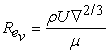(1)

Where ρ is density of water (1000 kg/m3), U is the inlet velocity, ∇ is the volume of the body (0.018 m3) and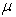is the viscosity of water (0.001 kg/m s).Download asVeiw figureFigures index
Figure 1. Hull design

### 3. Numerical Model

3.1. Mathematical Formulation

The considered calculation in this study is performed using basically the formulation of Reynolds Averaged Navier Stokes (RANS) equations [9, 10, 11] for an incompressible, isothermal and steady flow.

Equation of continuity: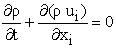(2)

Equations of motion: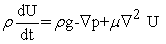(3)

Where U denotes the velocity vector,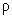is the mass density of water, p is the pressure, g is the acceleration of gravity andis the fluid dynamic viscosity.

In addition to the straight line investigation, the CFD analysis is carried out over different angle of incidence. Therefore, the use of a turbulence model [12, 13] which is capable to detect a separation zone becomes an imperative requirement. So, the numerical calculation is accomplished basing on two different turbulence models.

3.1.1. k-ε Model

As a good predictor of free stream flow, the formulations of k-ε turbulence model are expressed with the following equations: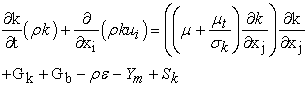(4)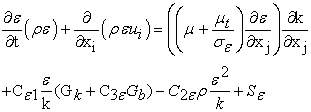(5)

Where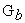denotes the generation of turbulent kinetic energy in terms of buoyancy,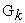mean the generation of turbulent kinetic energy in terms of velocity gradients.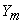represents the fluctuating dilation in compressible turbulence that contributes to the global dissipation rate.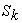and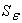are a user-defined sources.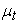is the turbulent viscosity defined with: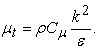(6)

The values of constant coefficients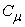,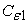,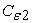,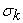and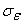are equal to 0.09, 1.44, 1.92, 1.0 and 1.3, respectively.

3.1.2. k-ω SST Model

As a developed turbulence model which provides a link between Reynolds Stresses and mean velocity, characterized by the following equations: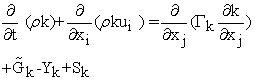(7)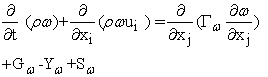(8)

Where,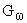represents the generation of ω,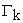and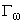represent the effective diffusivity of k and ω.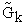represents the generation of turbulence kinetic energy due to mean velocity gradients.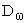represents the cross-diffusion term.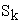and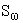are user-defined source terms.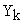and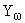represent the dissipation of k and ω due to turbulence.

The SST model combines the standard k-ε for more accurate near wall treatment with an automatic switch from a wall function to a low Re formulation based on grid spacing and the standard k-ε which is excellent in predicting the flow behavior in the free-stream.

The separation zone is predicted by the SST model while the k-ε fails to detect this zone. This is due to the lack of turbulent shear stress transportation in the k-ε model. This becomes extremely important with applications such as foils, as they are controlled by the flow separation profile and flow separation is formed by the transportation of turbulent shear stress.

3.2. Computational Domain

The fluid domain of this problem is specified with uniform inlet velocity varied between 0.4 m/s to 1.4 m/s for inlet and pressure outlet condition for outlet. The far field is defined as slip wall and finally the hull wall is defined with no slip condition taking into account the symmetric condition on the symmetry plane. For the analysis with strut arrangement, the establishment of the calculation is based on free flooding body modeling of the AUV hull relatively to captive model system as displayed in Figure 2.Download asVeiw figureFigures index
Figure 2. Computational domain with and without strut
3.3. Grid generation

The hybrid grid proves the worst mesh architecture using a structured topology for the close wall boundaries and unstructured gridding for far-field areas. To avoid the excessive number of hexahedral elements to mesh the far field boundary, the unstructured grid use a tetrahedral elements in this location. To detect the shear stress near the wall’s hull, the thickness of inflation layers is studied as displayed in Figure 3.Download asVeiw figureFigures index
Figure 3. Mesh

3.3.1. Boundary Layer

Basically, the thickness of boundary layers  is predicted using:(9)

The boundary layer thickness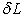can be estimated with the following empirical expression: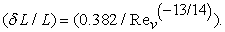(10)

Numerical studies are carried as an inspection of adequate y+ value in range between 1 to 4 using an increasing scale equal to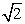as recommended by Eca et al. . In the other hand, the computational domain independency is mainly required in order to perform the calculation with an adequate precision. As presented in Table 1, the study is led to adapt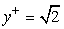for the computational domain 25DX6DX3D are the suitable grid features for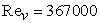(v= 1.4 m/s).

#### Table 1. Cd calculation for different y+

3.3.2. Meshing Independence

The study of mesh density is led to adapt Mesh 2 as a medium choice with no exceeded cell number and provides a result closed to the experimental one, as displayed in Table 2. In this case, the resulted drag coefficient is closed to experimental value.

### 4. Results and Discussion

4.1. Drag Coefficient for α=0°

The referred velocities of demonstration are described in Figure 4 with the variation of Reynolds number ranging from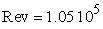to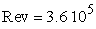within the straight line direction and for a depth H=4d. The CFD analysis is performed using two different turbulence models; k-ω SST and standard k-ε ,in order to select the best predictor of the hydrodynamic drag. According to these results, we can distinguish the decrease of the drag in regards of the Reynolds number increase. In this case, the flow still ever within the transition regime with a Reynolds number below 106. The fully turbulence model SST shows a respectable capabilities to follow the general tendencies of experimental results. This could be explained by the density of grid closed to the hull wall which stimulates the aptitude to detect the low Reynolds number streams around the model. Also, the standard k-ε model as well established model for low Reynolds problem revealed results in good agreement with experimental data. Also, it has been noted the light difference between single and two phase results with the current distance from water surface what proved the inconsiderable effect of free surface for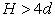with a straight line analysis.Download asVeiw figureFigures index
Figure 4.Variation of the Drag coefficient Cd vs Rev
4.2. Influence of the Angle of Attack

The hydrodynamic drag of the AUV hull is examined at four consecutive static angles of attack which are equal toα=0°, α=5°, α=10° and α=15° at the maximum flow speed of 1.4 m/s. As featured in Figure 5, the two different TCM show a good trend of following track of experimental results. But, it can be clearly seen that the standard k-ε turbulence model is more accurate in prediction of the drag coefficient over various angles of attack than the k-ω SST model which is unless efficient for low incidence level.

The over prediction of k-ω SST especially with α=15° is basically due to the over detection of flow separation occurred closer to the bow, then enhancing the axial drag and reducing the lift and side forces. According to k-ω SST model results, the separation point appears from α=5° at the R number of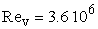what means that the hull is surrounding with fully laminar boundary layers on straight line test. In the other hand, the increase of the angle of attack for a fixed submerged depth of the vehicle, promotes the sensibility to the free surface and stimulates the disturbance around the tail section.Download asVeiw figureFigures index
Figure 5. Variation of the Drag coefficient Cd vs α (AOA)
4.3. Flow Separation

Although the over prediction of hydrodynamic resistance of k-ω SST model, it has been noted that the efficiency of this TCM in detect of the flow transition regime featured in Figure 6. The sliding of boundary layers is recognized with the variation of turbulent viscosity rate which increases according to the angle of attack. Therefore, the region of the onset of flow separation moves forward to the bow part and does not exceed the front half of the hull for α=15°. This factproves the avoidance of disturbance in this region for an extreme incidence. Also, the developed wake at the trailing edge grows as α increase due to the level of shearing stress affecting the inflation layers.Download asVeiw figureFigures index
Figure 6. Turbulence viscosity vs α
4.4. Effect of Free Surface

Figure 7 illustrates the calculation of pressure, viscous and total drag coefficients for various angles of attack in order to investigate the behavior of flow near the free surface. As the aft body approaches to the free surface, the pressure coefficient increases while the skin friction decreases especially below α=5°. The total drag is shown in good agreement with experimental values unlike a slight difference, what proves the effect of free surface on drag force variation. These results are confirmed by the distribution of the water volume fraction displayed in Figure 8. These results present the free surface water profiles developed at flow speed of 1.4 m/s, over various AOA and relative submerged depth of 4d. As can be seen, the disturbance and wave amount is become more important as the angle of attack increases and the tail section part approaching to the free surface, which stimulates the amount of pressure coefficient around this region.Download asVeiw figureFigures index
Figure 7. Variation of the Drag coefficient Cd vs α (AOA)Download asVeiw figureFigures index
Figure 8. Water volume fraction vs α (AOA)
4.5. Effect of Strut

A practicable development of the numerical analysis required to include the strut arrangement and properly represented the experimental facility. The bare hull is modulated as a free flooding body, and the investigation is performed with velocity of 1.4 m/s and various values of α. In addition, the direct comparison of CFD results with experimental data proves the effect of strut on predicted amount of drag force. In Figure 9, all three different results have the same trend according to the variation of angle of attack. However, the CFD results with strut show the best agreement with experimental measurements in front of the results without strut especially for high incidence. So, the effect of strut is proved and the negligence of its drag coefficient can affect the precision of numerical results taking into account that the difference between the two modes of investigation can attempt 10 % for α=15°.Download asVeiw figureFigures index
Figure 9. Variation of the Drag coefficient Cd with and without strut vs α (AOA)

### 5. Conclusions

In the current paper, we established an investigation about several factor neglected in some literature studies which can relatively affect the precision of such numerical analysis. The numerical analysis using the standard k-ε and k-ω SST turbulence models were compared with the experimental results obtained from towing tank facility. The results revealed that the standard k-ε is more efficient to predict the drag coefficient for various depth and different AOA. However, the k-ω SST proves a good capability to detect the region of the beginning of flow separation which is mainly important in establishing the sonar array. In the other hand, the modeling of experimental arrangement as a free flooding body including the strut in numerical calculation give a results in good agreement with experiment ones better than neglecting the effect of strut on drag coefficient. Also, the free surface shows a respectable sensitivity to AUV deviation which affects especially the predicted drag force.

In the future, we propose to develop a detailed investigation about the effect of strut design on the measurement of drag force in order to select the adequate arrangement.

### Acknowledgments

We are very appreciate to Laboratory of Electro-Mechanic Systems (LASEM) in the National School of Engineers of Sfax (ENIS( in Tunisia for providing the CPU time required for the current numerical analysis.

### References

  De Barros, E.A., Pascoal, A.M. and de Sa, E. Investigation of a method for predicting AUV derivatives. Ocean Engineering, Vol. 35, No. 16, pp. 1627-1636 (2008).In article View Article  Phillips, A., Furlong, M. and Turnock, S. The use of computational fluid dynamics to determine the dynamic stability of an autonomous underwater vehicle. In 10th Numerical Towing Tank Symposium (NuTTS’07). Hamburg, Germany, p. 6 (2007).In article PubMed  Tyagi, A. and Sen, D. Calculation of transverse hydrodynamic coefficients using computational fluid dynamic approach. Ocean Engineering, Vol. 33, No. 5-6, pp. 798-809 (2006).In article View Article  Stevenson, P., Furlong, M. and Dormer, D. AUV shapes - combining the practical and hydrodynamic considerations. OCEANS 2007-Europe. pp. 1-6 (2007).In article  Azarsina, F. and Williams, C.D. “Manoeuvring simulation of the MUN Explorer AUV based on the empirical hydrodynamics of axi-symmetric bare hulls”. Applied Ocean Research, Vol. 32, No. 4, pp. 443-453. (2010).In article View Article  Jagadeesh, P., Murali, K., Idichandy, V.G., Experimental investigation of hydrodynamic force coefficients over AUV hull form. Ocean Eng., 36 (2009). 113-118.In article View Article  Dantas, J.L.D. and Barros, E.A. Numerical Analysis of Control Surface Effects on AUV Maneuverability. Applied Ocean Research, 42 (2013), 168-181.In article View Article  Myring, D.F. “A theoretical study of body drag in subcritical axisymmetric flow”. Aeronautical Quartely, Vol. 27, No. 3, 27 (1976).In article View Article  ANSYS. ANSYS FLUENT theory guide. (Canonsburg, PA: USA), pp. 114-115. (2009).In article  White, F.M. Viscous Fluid Flow, (third edition University of Rhode, Island), pp. 395-396 (2006).In article  Versteeg, H., M., and Malalasekera, W. An Introduction to Computational Fluid dynamics: The Finite Volume Method, Longman Scientific and Technical, U.K (1995).In article  Menter, F., R., 1994. Two-equation eddy-viscosity turbulence models for engineering applications. AIAA Journal, 32, 1598-1605.In article View Article  Sarkar, T., Sayer, P., G., Fraser, S., M. Study of Autonomous Underwater Vehicle Hull forms using Computational Fluid Dynamics. International Journal for Numerical Methods in Fluids. 25(1997), 1301-1313.In article View Article  Schlichting, H., 1966. Boundary Layer Theory, McGraw- Hill, New York.In article  Eca L., Vaz G., Hoekstra M. A verification and validation exercise for the flow over a backward facing step. In: ECCOMAS CFD –V Eur. conf. on comput. fluiddyn., Lisbon, Portugal. 2010.In article PubMed